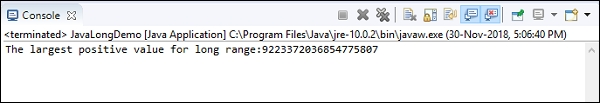# What is the equivalent of Java long in the context of MySQL variables?

The equivalent of Java long in the context of MySQL variables is BigInt.

In Java, the long datatype takes 8 bytes while BigInt also takes the same number of bytes.

### Demo of Java long

Here is the demo of Java long −

public class JavaLongDemo {
public static void main(String[]args) {
long kilometer = 9223372036854775807L;
System.out.println("The largest positive value for long range:"+kilometer);
}
}

The following is the output −### Demo of BigInt

Let us see an example of BigInt type in MySQL. The following is the query to create a table with field set as BigInt −

mysql> create table BigIntDemo
−> (
−> Kilometer BigInt
−> );
Query OK, 0 rows affected (0.74 sec)

Inserting some records in the table. The query is as follows −

mysql> insert into BigIntDemo values(9223372036854775807);
Query OK, 1 row affected (0.19 sec)

Now you can check whether the above record is inserted or not. The select query is as follows −

mysql> select *from BigIntDemo;

The following is the output −

+---------------------+
| Kilometer           |
+---------------------+
| 9223372036854775807 |
+---------------------+
1 row in set (0.00 sec)